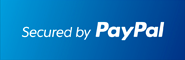** New: ECONOMICS , DIFFERENTIAL EQUATIONS **         ** SPECIALS for bulk apps **         ** Bestsellers: CALCULUS , SAT , PHYSICS , CHEM , STOCKS & PORTFOLIOS , ALGEBRA **# #1 Equation Solver Solver for the TI-89 Calculator

## Step by Step Equation Solver

### Works With TI92 Plus TI89 TI89 Titanium Voyage 200At the end of the PayPal checkout, you will be sent an email containing your key and software.Description

• Users have boosted their Algebra knowledge.
• Shows Step by Step solutions when
• Solving equations Step by Step : including exponentials, logarithms, absolute value, linear functions, quadratic functions, power functions, etc Solving Logarithmic equations of any base Step by Step
• Solving inequalities Step by Step
• Solving system of equations Step by Step
• Complex numbers Step by Step
• Synthetic fractions Step by Step
• Partial fractions Step by Step
• Rationalizing the denominator Step by Step
• Completing the square Step by Step
• Computing powers, roots Step by Step
• Solving Money problems Step by Step
• Factoring by Groups Step by Step
• My Equation Manager: Save and solve your own equations.
• and much more.
• Ideal for quick review and homework check.
• Easy to use. Just plug in and the correct answer shows.

Visit the Classic Site at www.ti89.com/index.htm for more details.

Documentation